# Problem_10_9_26.r
#   Problem 10.26 of Rice
#   Hampson and Walker data on heats of sublimation of
#       platinum,iridium, and rhodium

# To install these packages, uncomment the next two lines
#install.packages("MASS")
#install.packages("boot")

library(MASS)
## Warning: package 'MASS' was built under R version 3.1.3
library(boot)
## Warning: package 'boot' was built under R version 3.1.3
#
x.platinum=scan(file="Rice 3e Datasets/ASCII Comma/Chapter 10/platinum.txt")
x.iridium=scan(file="Rice 3e Datasets/ASCII Comma/Chapter 10/iridium.txt")
x.rhodium=scan(file="Rice 3e Datasets/ASCII Comma/Chapter 10/rhodium.txt")

# Parts (a)-(d)
x=x.platinum
par(mfcol=c(2,2))
hist(x,main="Platinum")
stem(x)
##
##   The decimal point is at the |
##
##   132 | 7
##   134 | 134578889900224488
##   136 | 36
##   138 |
##   140 | 2
##   142 | 3
##   144 |
##   146 | 58
##   148 | 8
boxplot(x)
plot(x)

x=x.rhodium
par(mfcol=c(2,2))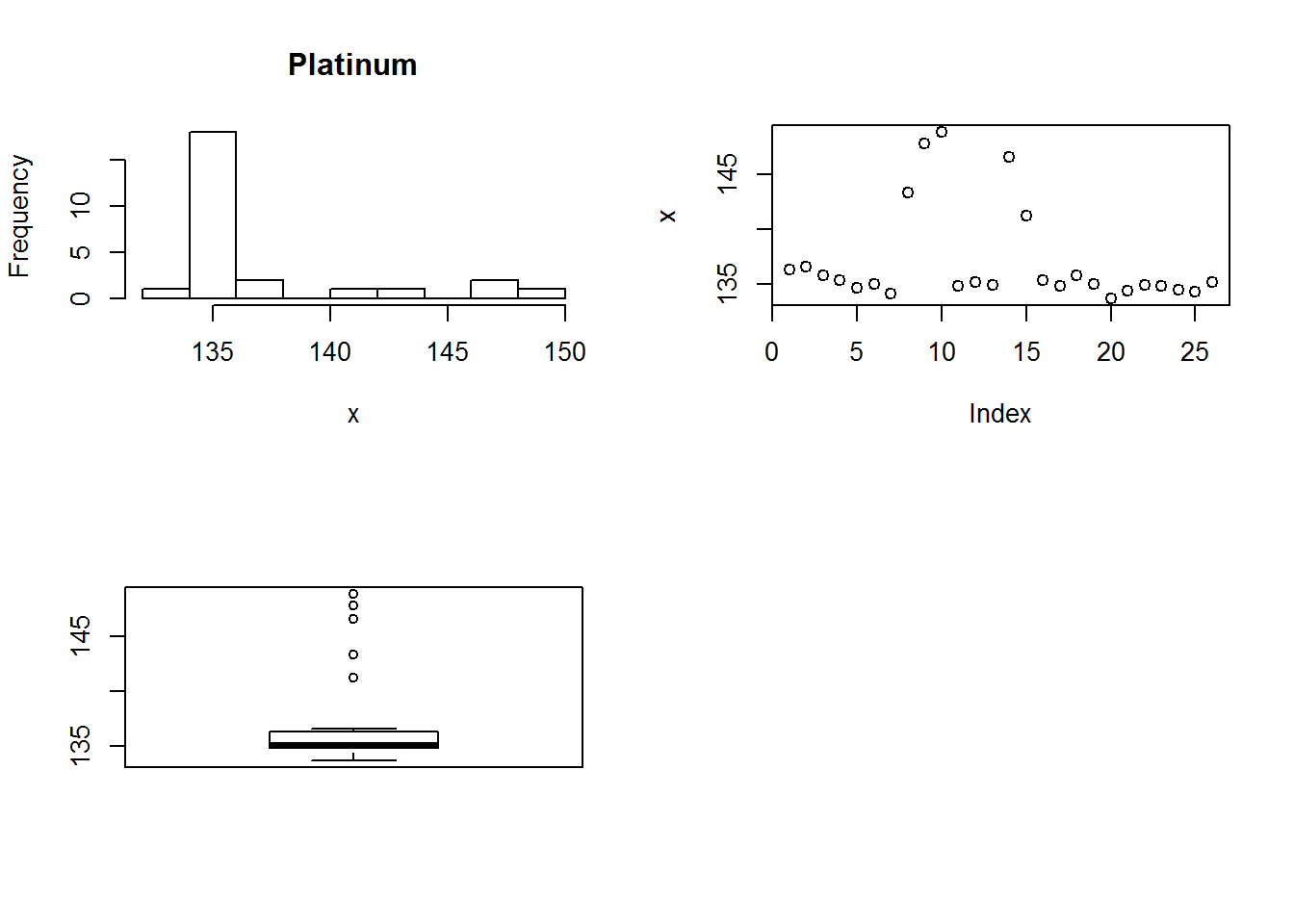hist(x,main="Rhodium")
stem(x)
##
##   The decimal point is at the |
##
##   126 | 4
##   127 |
##   128 |
##   129 |
##   130 |
##   131 | 111112234569
##   132 | 123456677899
##   133 | 000333455558
##   134 | 12
##   135 | 7
boxplot(x)
plot(x)

x=x.iridium
par(mfcol=c(2,2))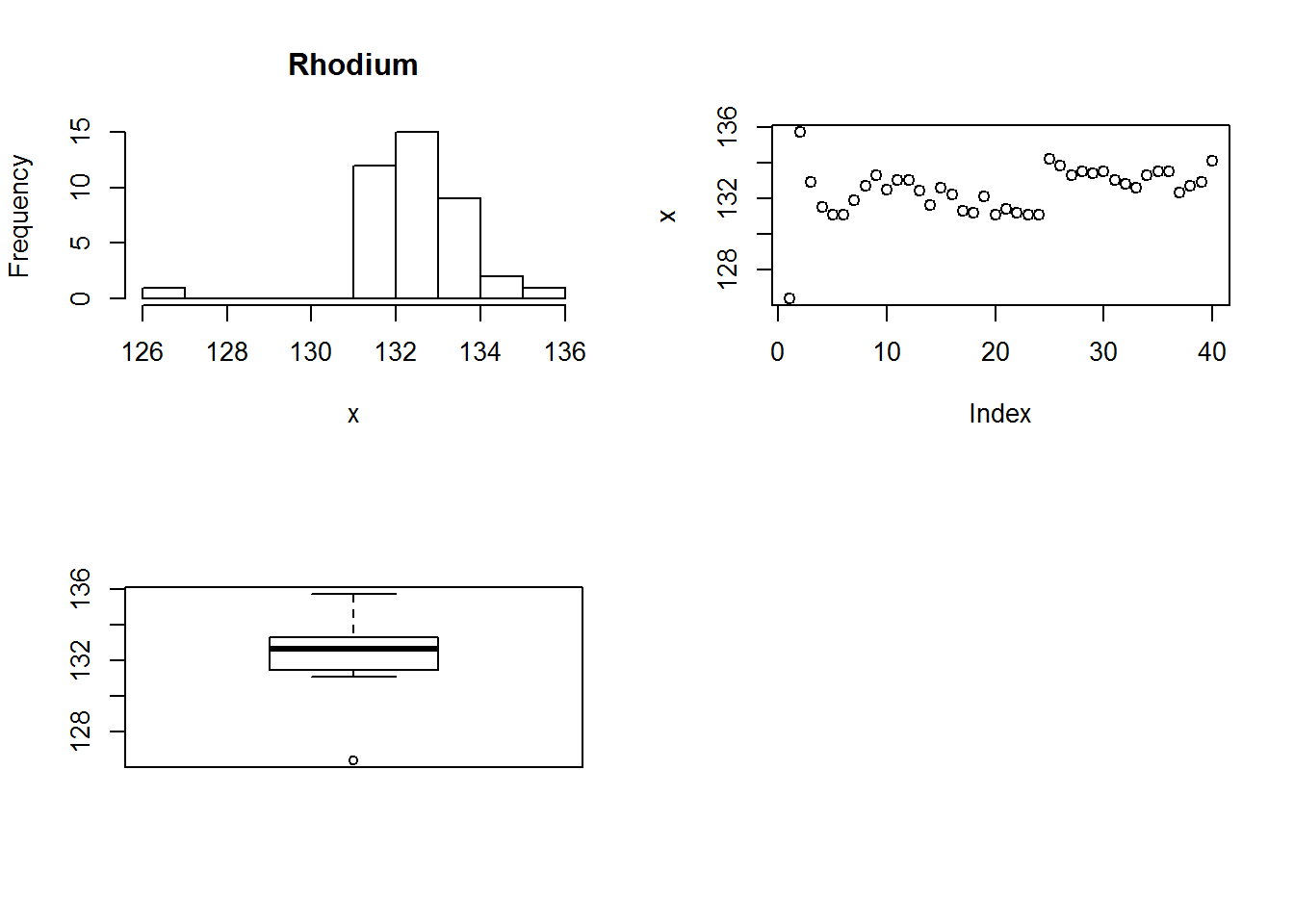hist(x,main="Iridium")
stem(x)
##
##   The decimal point is 1 digit(s) to the right of the |
##
##   13 | 7
##   14 |
##   14 | 5
##   15 | 2
##   15 | 999
##   16 | 00000000000000001113
##   16 |
##   17 | 4
boxplot(x)
plot(x)
plot(c(10:length(x)), x[10:length(x)], main="Iridium")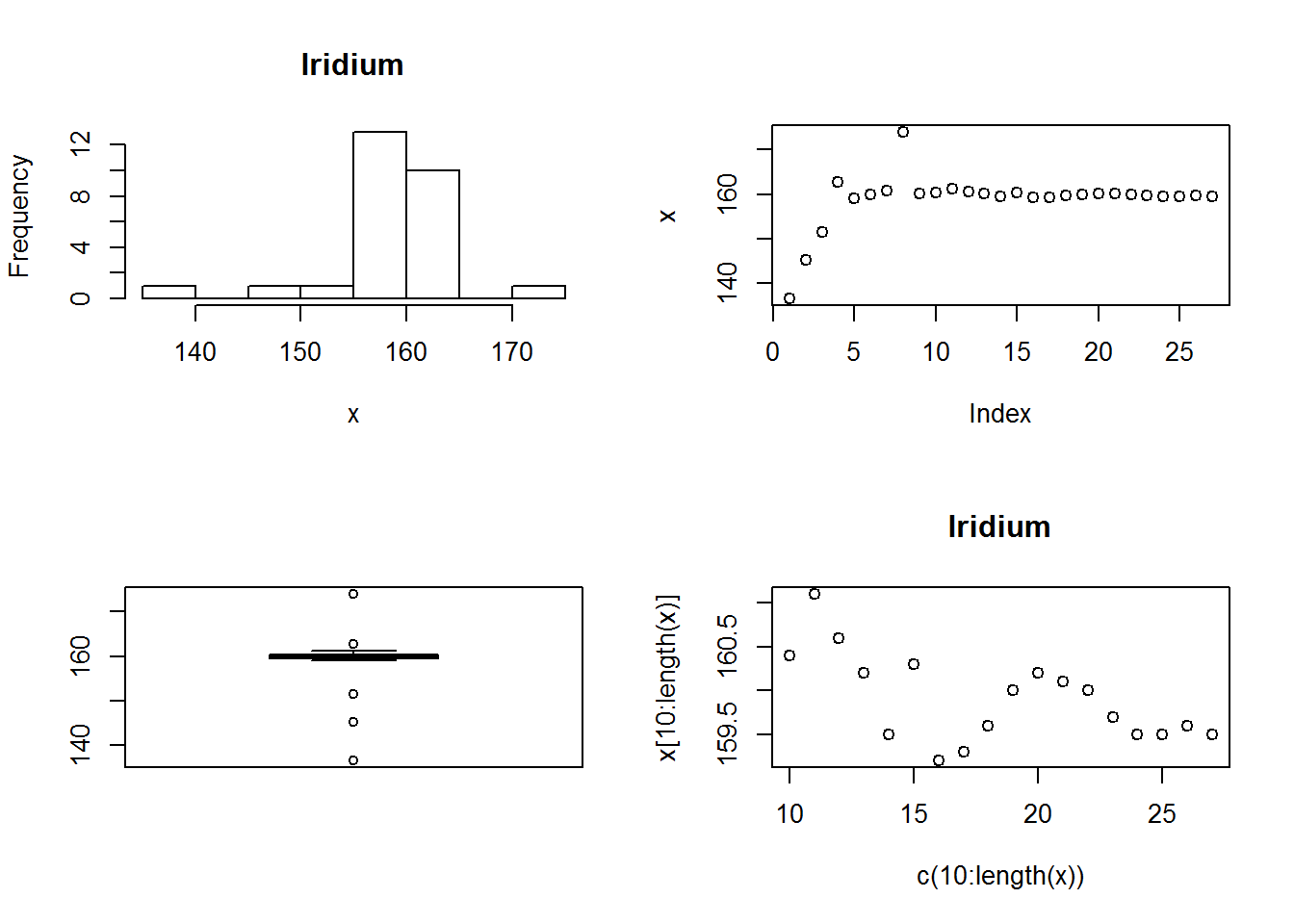# (e) For Platinum, observations 8,9,10, and 14,5 seem much higher than the rest.
#     They do not seem iid

# (e) For Rhodium,  the first 2 observations are very variable (low then high).
#  and the observations after about number 25 seem different from those before.
#  These data do not seem iid either.

# (e) For Iridium,  the first 4 observations steadily increase and observaton 8 seems much higher
# than the rest.  These data do not appear iid. Also, plotting the observations from 10 on,
# there seems to be time series dependence -- closer observations in the sequence have more similar
# values.

# (f) Measures of location
#   For Rhodium
x=x.rhodium
mean(x); mean(x,trim=.1);mean(x,trim=.2);median(x); huber(x,k=1.5)[]
##  132.42
##  132.4781
##  132.5292
##  132.65
##  132.4921
# All the estimates are very close
# The mean is the lowest, it is affected by the lowest value
# The trimmed means exclude the extreme values and are similar to the median.

#   For Iridium
x=x.iridium
mean(x); mean(x,trim=.1);mean(x,trim=.2);median(x); huber(x,k=1.5)[]
##  158.8148
##  159.5478
##  159.8412
##  159.8
##  159.8545
# The mean is much lower than the other estimates
# The mean is the lowest, it is affected by the lowest value
# The trimmed means exclude the extreme values and are similar to the median.

# (g) Standard error of the sample mean and approximate 90 percent conf. interval
# First for rhodium:
x=x.rhodium
x.stdev=sqrt(var(x))
x.mean.sterr=x.stdev/sqrt(length(x))

print(x.mean.sterr)
##  0.2273369
alpha=0.10
z.upperalphahalf=qnorm(1-alpha/2)
x.mean=mean(x)
x.mean.ci<-c(-1,1)*z.upperalphahalf* x.mean.sterr + x.mean
print(x.mean); print(x.mean.ci)
##  132.42
##  132.0461 132.7939
stats1.rhodium=c(x.mean, x.mean.ci)

# Second for iridium
x=x.iridium
x.stdev=sqrt(var(x))
x.mean.sterr=x.stdev/sqrt(length(x))

print(x.mean.sterr)
##  1.197917
alpha=0.10
z.upperalphahalf=qnorm(1-alpha/2)
x.mean=mean(x)
x.mean.ci<-c(-1,1)*z.upperalphahalf* x.mean.sterr + x.mean
print(x.mean); print(x.mean.ci)
##  158.8148
##  156.8444 160.7852
stats1.iridium=c(x.mean, x.mean.ci)

# parts (i), (j), (k).
# Bootstrap confidence intervals of different location measures

fcn.median<- function(x, d) {
return(median(x[d]))
}
fcn.mean<- function(x, d) {
return(mean(x[d]))
}

fcn.trimmedmean <- function(x, d, trim=0) {
return(mean(x[d], trim/length(x)))
}

fcn.huber<-function(x,d){
x.huber=huber(x[d],k=1.5)
return(x.huber[])
}

#  First for rhodium
set.seed(1)
x=x.rhodium

# Bootstrap analysis of sample 20% trimmed mean:
x.boot.trimmedmean.2= boot(x, fcn.trimmedmean,trim=.2, R=1000)
par(mfcol=c(1,2))
plot(x.boot.trimmedmean.2)
title(paste("Trimmed Mean:  StDev=",as.character(round(sqrt(var(x.boot.trimmedmean.2$t)),digits=4)),sep=""), adj=1)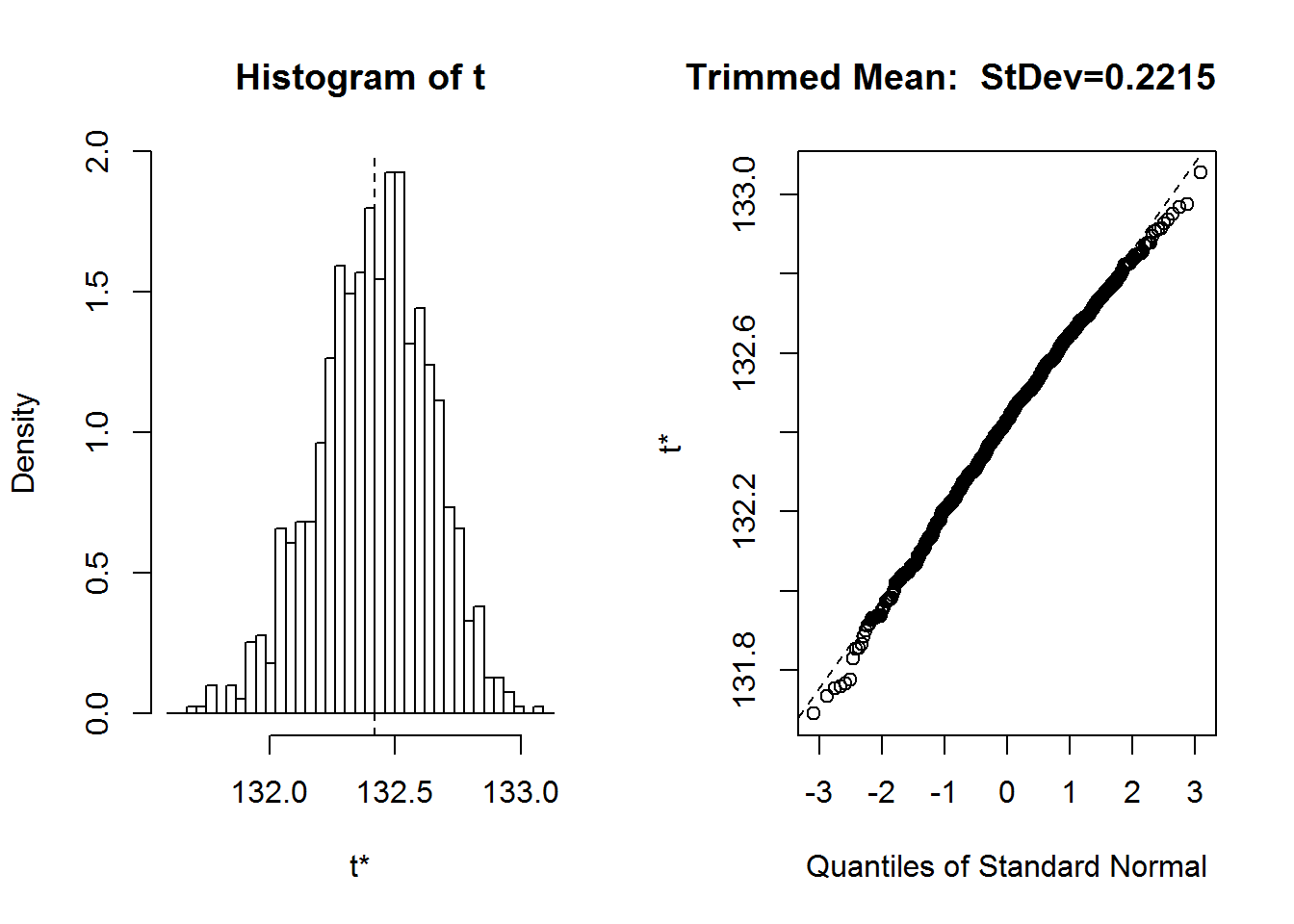print(x.boot.trimmedmean.2$t0)
##  132.42
print(sqrt(var(x.boot.trimmedmean.2$t))) ## [,1] ## [1,] 0.2214742 stats2.trim20.rhodium<-c(x.boot.trimmedmean.2$t0,(sqrt(var(x.boot.trimmedmean.2$t)))) # boot.ci(x.boot.trimmedmean.2,conf=.90, type="basic") ## BOOTSTRAP CONFIDENCE INTERVAL CALCULATIONS ## Based on 1000 bootstrap replicates ## ## CALL : ## boot.ci(boot.out = x.boot.trimmedmean.2, conf = 0.9, type = "basic") ## ## Intervals : ## Level Basic ## 90% (132.1, 132.8 ) ## Calculations and Intervals on Original Scale # Bootstrap analysis of sample 10% trimmed mean: x.boot.trimmedmean.1= boot(x, fcn.trimmedmean,trim=.1, R=1000) par(mfcol=c(1,2)) plot(x.boot.trimmedmean.1) title(paste("Trimmed Mean: StDev=",as.character(round(sqrt(var(x.boot.trimmedmean.1$t)),digits=4)),sep=""),
adj=1)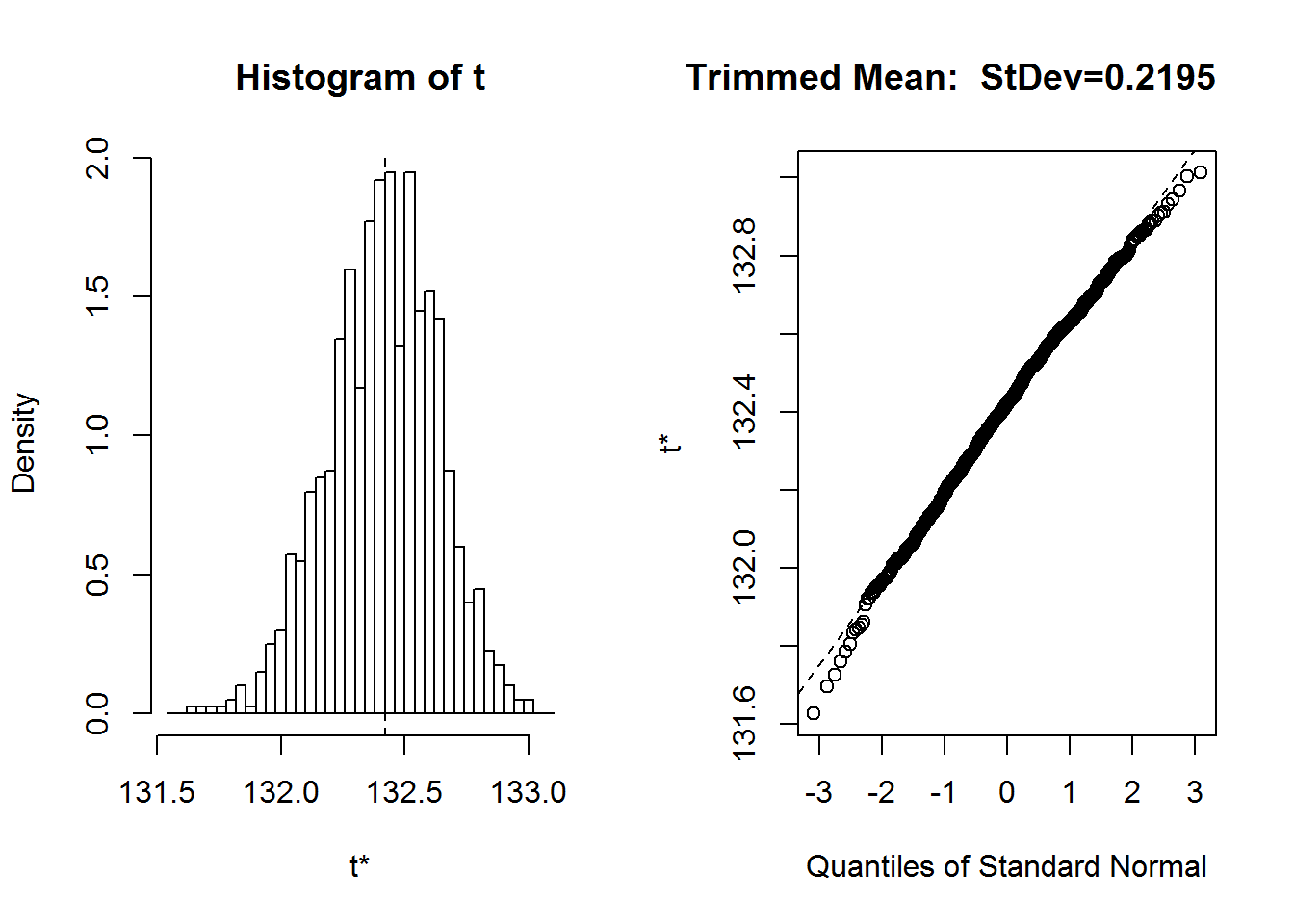print(x.boot.trimmedmean.1$t0) ##  132.42 print(sqrt(var(x.boot.trimmedmean.1$t)))
##           [,1]
## [1,] 0.2195023
stats2.trim10.rhodium<-c(x.boot.trimmedmean.1$t0,(sqrt(var(x.boot.trimmedmean.1$t))))
#
#
boot.ci(x.boot.trimmedmean.1,conf=.90, type="basic")
## BOOTSTRAP CONFIDENCE INTERVAL CALCULATIONS
## Based on 1000 bootstrap replicates
##
## CALL :
## boot.ci(boot.out = x.boot.trimmedmean.1, conf = 0.9, type = "basic")
##
## Intervals :
## Level      Basic
## 90%   (132.1, 132.8 )
## Calculations and Intervals on Original Scale
# Bootstrap analysis of sample median:
x.boot.median= boot(x, fcn.median, R=1000)
plot(x.boot.median)
title(paste("Median:  StDev=",as.character(round(sqrt(var(x.boot.median$t)),digits=4)),sep=""), adj=1)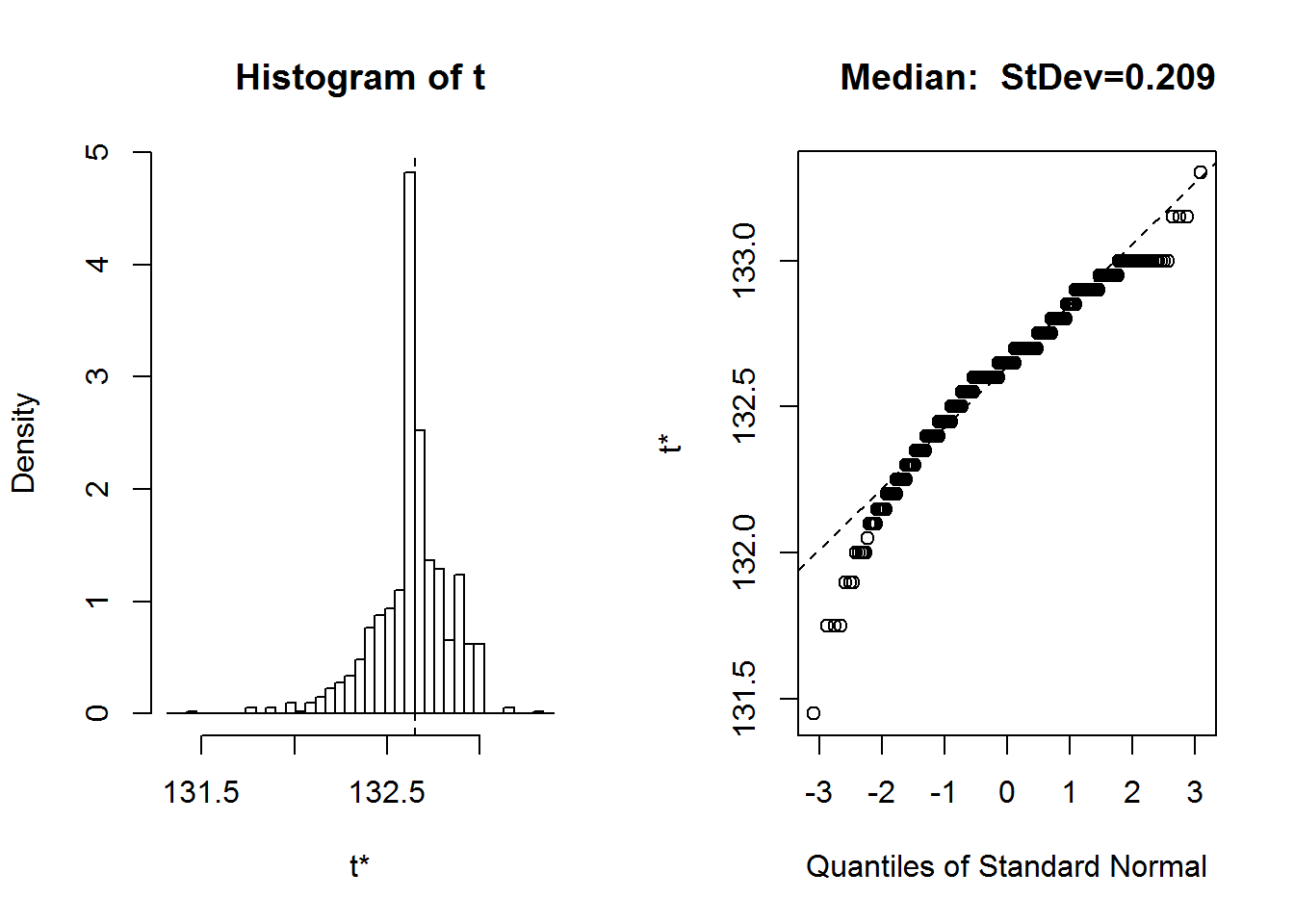print(x.boot.median$t0)
##  132.65
print(sqrt(var(x.boot.median$t))) ## [,1] ## [1,] 0.2090489 boot.ci(x.boot.median,conf=.90, type="basic") ## BOOTSTRAP CONFIDENCE INTERVAL CALCULATIONS ## Based on 1000 bootstrap replicates ## ## CALL : ## boot.ci(boot.out = x.boot.median, conf = 0.9, type = "basic") ## ## Intervals : ## Level Basic ## 90% (132.3, 133.0 ) ## Calculations and Intervals on Original Scale stats2.median.rhodium<-c(x.boot.median$t0,(sqrt(var(x.boot.median$t)))) stats1.rhodium ##  132.4200 132.0461 132.7939 # The bootstrap estimate and standard errrors are given by: stats2.trim20.rhodium ##  132.4200000 0.2214742 stats2.trim10.rhodium ##  132.4200000 0.2195023 stats2.median.rhodium ##  132.6500000 0.2090489 # For Rhodium these are all about the same # (k). The approximate 90% confidence intervals are: boot.ci(x.boot.trimmedmean.2,conf=.90, type="basic") ## BOOTSTRAP CONFIDENCE INTERVAL CALCULATIONS ## Based on 1000 bootstrap replicates ## ## CALL : ## boot.ci(boot.out = x.boot.trimmedmean.2, conf = 0.9, type = "basic") ## ## Intervals : ## Level Basic ## 90% (132.1, 132.8 ) ## Calculations and Intervals on Original Scale boot.ci(x.boot.trimmedmean.1,conf=.90, type="basic") ## BOOTSTRAP CONFIDENCE INTERVAL CALCULATIONS ## Based on 1000 bootstrap replicates ## ## CALL : ## boot.ci(boot.out = x.boot.trimmedmean.1, conf = 0.9, type = "basic") ## ## Intervals : ## Level Basic ## 90% (132.1, 132.8 ) ## Calculations and Intervals on Original Scale boot.ci(x.boot.median,conf=.90, type="basic") ## BOOTSTRAP CONFIDENCE INTERVAL CALCULATIONS ## Based on 1000 bootstrap replicates ## ## CALL : ## boot.ci(boot.out = x.boot.median, conf = 0.9, type = "basic") ## ## Intervals : ## Level Basic ## 90% (132.3, 133.0 ) ## Calculations and Intervals on Original Scale # These are all essential equal for rhodium # These intervals are all about the same as that based on part (g) stats1.rhodium ##  132.4200 132.0461 132.7939 # ############## # Second for iridium set.seed(1) x=x.iridium # Bootstrap analysis of sample 20% trimmed mean: x.boot.trimmedmean.2= boot(x, fcn.trimmedmean,trim=.2, R=1000) par(mfcol=c(1,2)) plot(x.boot.trimmedmean.2) title(paste("Trimmed Mean: StDev=",as.character(round(sqrt(var(x.boot.trimmedmean.2$t)),digits=4)),sep=""),
adj=1)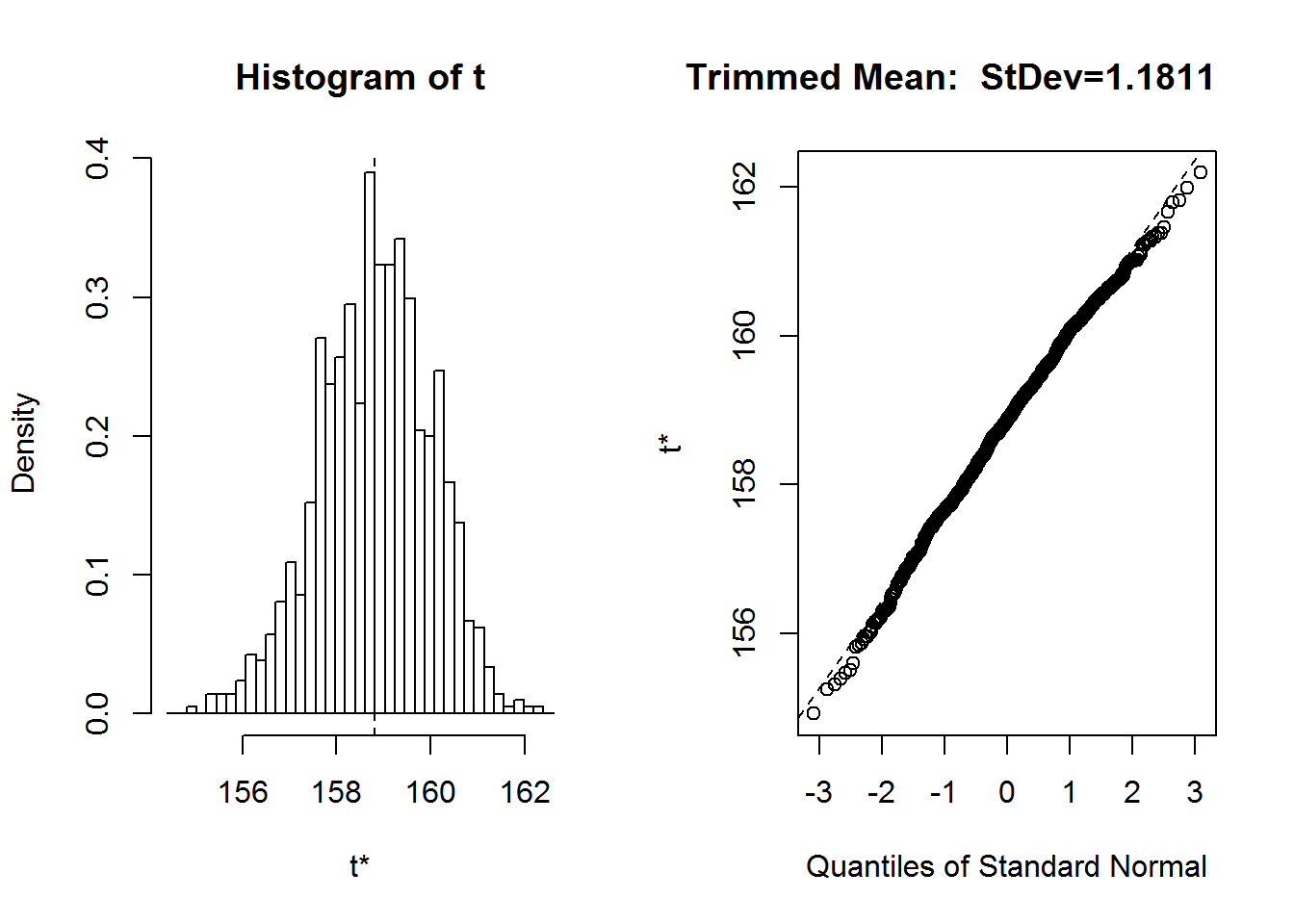print(x.boot.trimmedmean.2$t0) ##  158.8148 print(sqrt(var(x.boot.trimmedmean.2$t)))
##          [,1]
## [1,] 1.181086
stats2.trim20.iridium<-c(x.boot.trimmedmean.2$t0,(sqrt(var(x.boot.trimmedmean.2$t))))
#
boot.ci(x.boot.trimmedmean.2,conf=.90, type="basic")
## BOOTSTRAP CONFIDENCE INTERVAL CALCULATIONS
## Based on 1000 bootstrap replicates
##
## CALL :
## boot.ci(boot.out = x.boot.trimmedmean.2, conf = 0.9, type = "basic")
##
## Intervals :
## Level      Basic
## 90%   (157.0, 160.8 )
## Calculations and Intervals on Original Scale
# Bootstrap analysis of sample 10% trimmed mean:
x.boot.trimmedmean.1= boot(x, fcn.trimmedmean,trim=.1, R=1000)
par(mfcol=c(1,2))
plot(x.boot.trimmedmean.1)
title(paste("Trimmed Mean:  StDev=",as.character(round(sqrt(var(x.boot.trimmedmean.1$t)),digits=4)),sep=""), adj=1)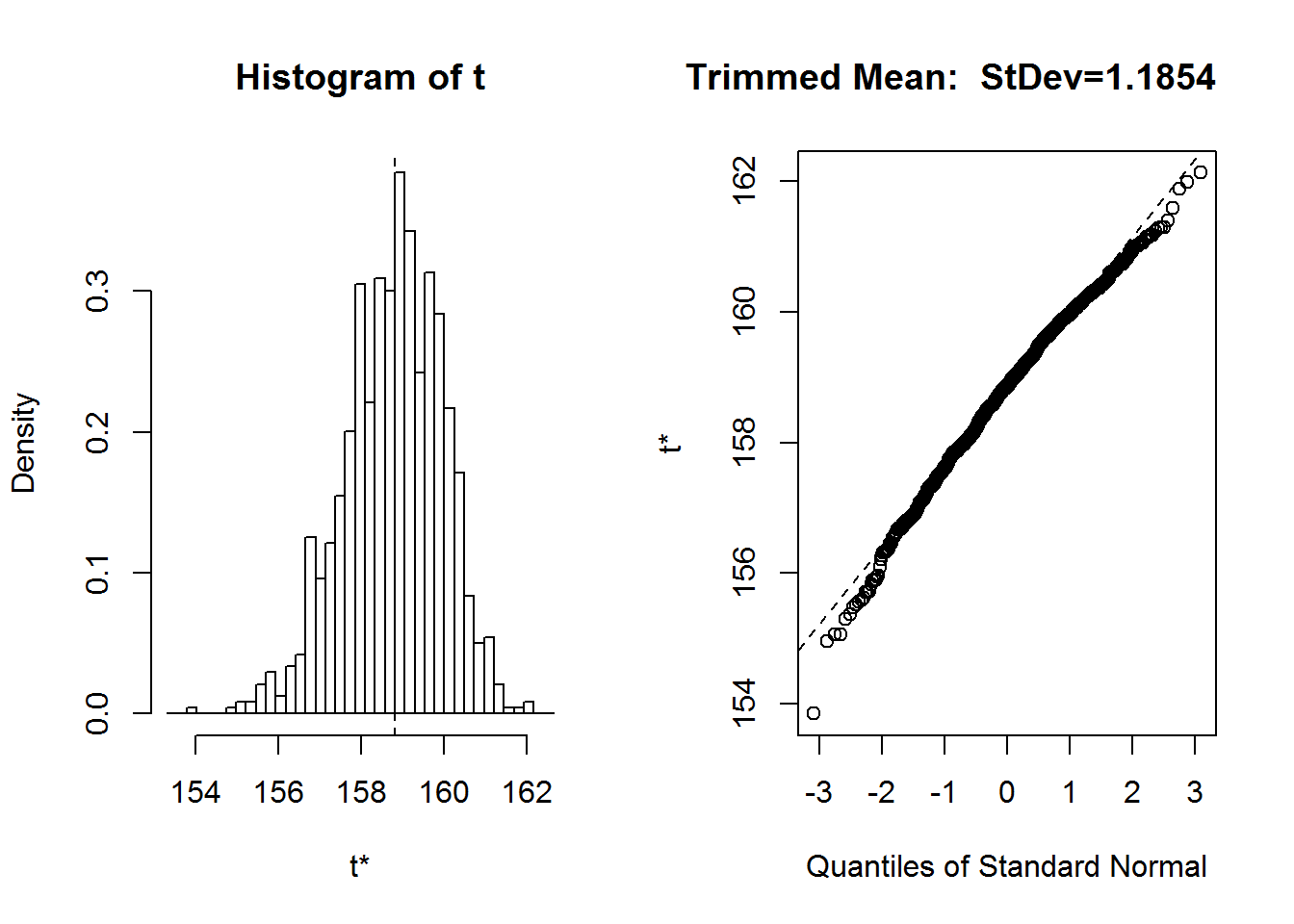print(x.boot.trimmedmean.1$t0)
##  158.8148
print(sqrt(var(x.boot.trimmedmean.1$t))) ## [,1] ## [1,] 1.185398 stats2.trim10.iridium<-c(x.boot.trimmedmean.1$t0,(sqrt(var(x.boot.trimmedmean.1$t)))) # # boot.ci(x.boot.trimmedmean.1,conf=.90, type="basic") ## BOOTSTRAP CONFIDENCE INTERVAL CALCULATIONS ## Based on 1000 bootstrap replicates ## ## CALL : ## boot.ci(boot.out = x.boot.trimmedmean.1, conf = 0.9, type = "basic") ## ## Intervals : ## Level Basic ## 90% (157.0, 160.9 ) ## Calculations and Intervals on Original Scale # Bootstrap analysis of sample median: x.boot.median= boot(x, fcn.median, R=1000) plot(x.boot.median) title(paste("Median: StDev=",as.character(round(sqrt(var(x.boot.median$t)),digits=4)),sep=""),
adj=1)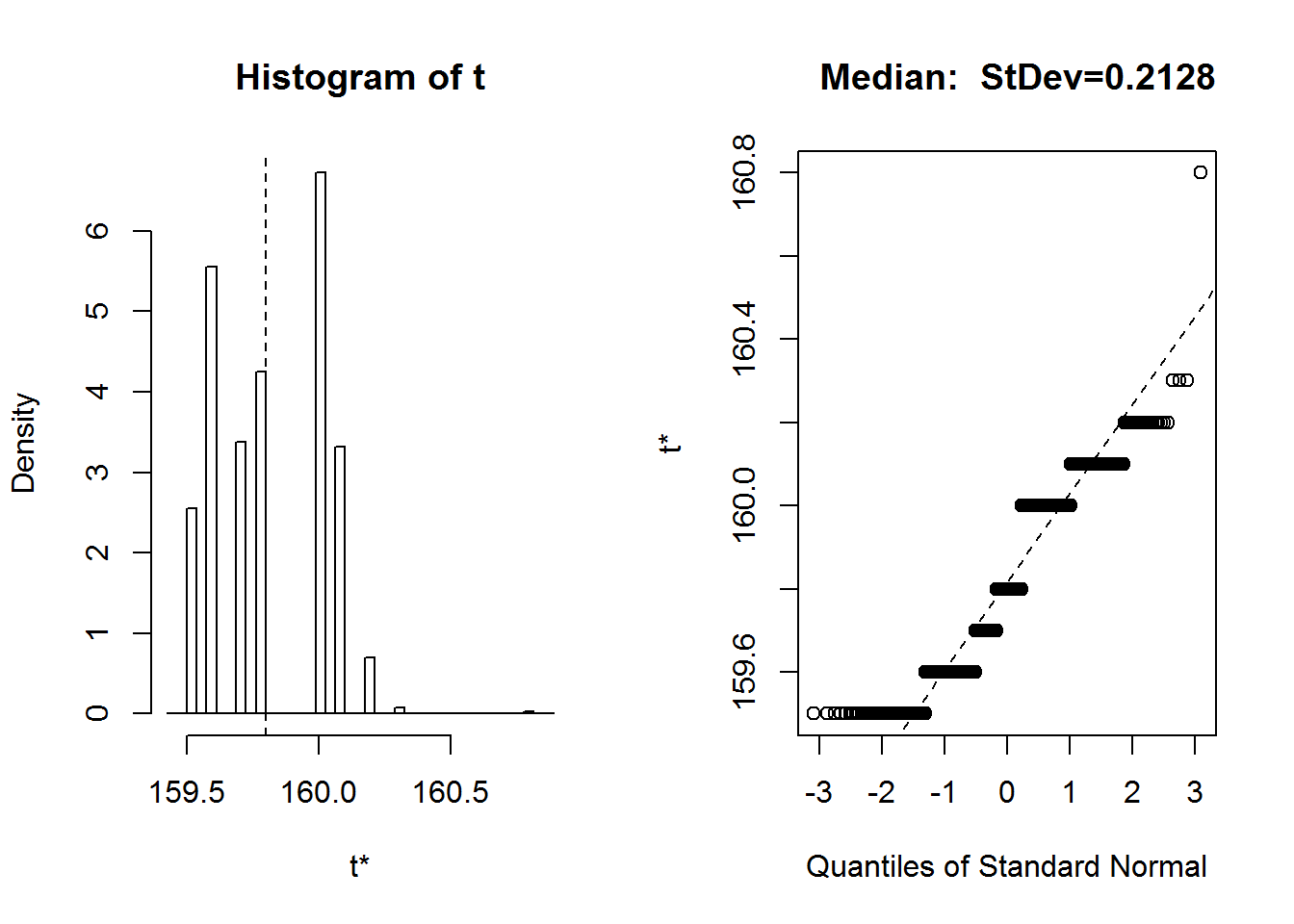print(x.boot.median$t0) ##  159.8 print(sqrt(var(x.boot.median$t)))
##           [,1]
## [1,] 0.2127957
boot.ci(x.boot.median,conf=.90, type="basic")
## BOOTSTRAP CONFIDENCE INTERVAL CALCULATIONS
## Based on 1000 bootstrap replicates
##
## CALL :
## boot.ci(boot.out = x.boot.median, conf = 0.9, type = "basic")
##
## Intervals :
## Level      Basic
## 90%   (159.5, 160.1 )
## Calculations and Intervals on Original Scale
stats2.median.iridium<-c(x.boot.median$t0,(sqrt(var(x.boot.median$t))))

stats1.iridium
##  158.8148 156.8444 160.7852
stats2.trim20.iridium
##  158.814815   1.181086
stats2.trim10.iridium
##  158.814815   1.185398
stats2.median.iridium
##  159.8000000   0.2127957
##############
# The bootstrap estimate and standard errrors are given by:
stats2.trim20.iridium
##  158.814815   1.181086
stats2.trim10.iridium
##  158.814815   1.185398
stats2.median.iridium
##  159.8000000   0.2127957
# For iridium the median has much lower st error than the trimmed means

#  (k). The approximate 90% confidence intervals are:

boot.ci(x.boot.trimmedmean.2,conf=.90, type="basic")
## BOOTSTRAP CONFIDENCE INTERVAL CALCULATIONS
## Based on 1000 bootstrap replicates
##
## CALL :
## boot.ci(boot.out = x.boot.trimmedmean.2, conf = 0.9, type = "basic")
##
## Intervals :
## Level      Basic
## 90%   (157.0, 160.8 )
## Calculations and Intervals on Original Scale
boot.ci(x.boot.trimmedmean.1,conf=.90, type="basic")
## BOOTSTRAP CONFIDENCE INTERVAL CALCULATIONS
## Based on 1000 bootstrap replicates
##
## CALL :
## boot.ci(boot.out = x.boot.trimmedmean.1, conf = 0.9, type = "basic")
##
## Intervals :
## Level      Basic
## 90%   (157.0, 160.9 )
## Calculations and Intervals on Original Scale
boot.ci(x.boot.median,conf=.90, type="basic")
## BOOTSTRAP CONFIDENCE INTERVAL CALCULATIONS
## Based on 1000 bootstrap replicates
##
## CALL :
## boot.ci(boot.out = x.boot.median, conf = 0.9, type = "basic")
##
## Intervals :
## Level      Basic
## 90%   (159.5, 160.1 )
## Calculations and Intervals on Original Scale
# The interval using the median is much smaller than that for the trimmed means
# These intervals are all smaller than that based on part (g)
stats1.iridium
##  158.8148 156.8444 160.7852